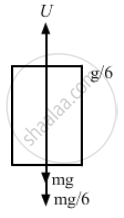# An Empty Plastic Box of Mass M is Found to Accelerate up at the Rate of G/6 When Placed Deep Inside Water. How Much Sand Should Be Put Inside the Box So that It May Accelerate Down at the Rate of G/6? - Physics

Sum

An empty plastic box of mass m is found to accelerate up at the rate of g/6 when placed deep inside water. How much sand should be put inside the box so that it may accelerate down at the rate of g/6?

#### Solution

Let U be the upward force of water acting on the plastic box.
Let m be the initial mass of the plastic box.
When the empty plastic box is accelerating upward,
$U - mg = \frac{mg}{6}$
$\Rightarrow U = \frac{7 mg}{6}$$\Rightarrow m = \frac{6U}{7g} . . . . \left( i \right)$
Let M be the final mass of the box after putting some sand in it.
$Mg - U = \frac{Mg}{6}$
$\Rightarrow Mg - \frac{Mg}{6} = U$
$\Rightarrow M = \frac{6U}{5g} . . . . \left( ii \right)$
$= \frac{6U}{5g} - \frac{6U}{7g}$
$= \frac{6U\left( 7 - 5 \right)}{35 g}$
$= \frac{6U \cdot 2}{35 g}$

From equation (i),
$m = \frac{6U}{7g}$
$= \frac{2}{5}m$
Concept: Newton’s Second Law of Motion
Is there an error in this question or solution?

#### APPEARS IN

HC Verma Class 11, Class 12 Concepts of Physics Vol. 1
Chapter 5 Newton's Laws of Motion
Q 20 | Page 80